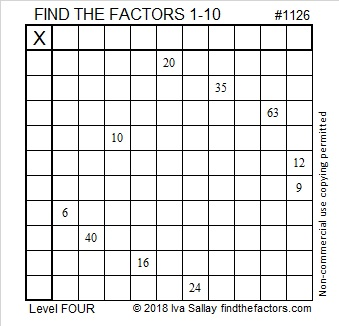# 1126 and Level 4

Since we are only using factors from 1 to 10, we have only one common factor of 12 and 9 to consider instead of two. Also, you will need to ask yourself, “Where is the only place ____ can fit in the first column (or the top row).” to solve this puzzle. Good Luck!Print the puzzles or type the solution in this excel file: 10-factors-1121-1133

Here are some facts about the number 1126:

• 1126 is a composite number.
• Prime factorization: 1126 = 2 × 563
• The exponents in the prime factorization are 1 and 1. Adding one to each and multiplying we get (1 + 1)(1 + 1) = 2 × 2 = 4. Therefore 1126 has exactly 4 factors.
• Factors of 1126: 1, 2, 563, 1126
• Factor pairs: 1126 = 1 × 1126 or 2 × 563
• 1126 has no square factors that allow its square root to be simplified. √1126 ≈ 33.55592I chuckled when I noticed that the first five digits of √1126 are all the factors in 1125’s prime factorization.

1126 is palindrome 1K1 in BASE 25 (K is 20 base 10) because 25² + 20(25) + 1 = 1126

This site uses Akismet to reduce spam. Learn how your comment data is processed.